67 Rates

# Viscosity and Viscometry

The physical quantity ‘viscosity’ gives information on how thick a fluid is and how easily it flows. In scientific terms, viscosity is the measure of a fluid’s internal flow resistance. It is the resistance which a fluid shows when being deformed.

This page focuses on how to define viscosity, the different types of viscosity, and the different types of liquids which show either Newtonian or non-Newtonian flow behavior. Viscosity is a physical parameter which is measured throughout industry to gain insight into materials and their behavior.

### Articles about viscosity

Discover more in-depth articles on viscosity and related topics:

### Viscosity tables

Finde useful viscosity tables to help you in your daily work:

### Viscometers

Anton Paar offers a wide range of viscometers to suit different applications and industrial requirements:

## How to define viscosity

In order to determine a fluid’s viscosity, you have to enter the field of viscometry, a subject area of a wider science called rheology. Rheology deals with the flow behavior and deformation of materials.

Imagine all materials as classified on a virtual scale from solid to liquid. Scientists specify solid materials as being elastic and liquids as being viscous. In everyday life, we mostly come across viscoelastic materials. That is, substances which are neither completely elastic, nor entirely viscous. According to a material’s properties, we either classify it as a viscoelastic solid (like e.g. sweet jelly) or as a viscoelastic liquid (like e.g. a yoghurt drink or shower gel).

The specific field of viscometry covers ideally viscous fluids, and – considering certain restrictions – also viscoelastic liquids, i.e. viscous fluids that contain an elastic portion. Fluids which flow easily show a low resistance to deformation. They are low-viscosity fluids. High-viscosity fluids resist deformation. Consequently, they do not flow easily.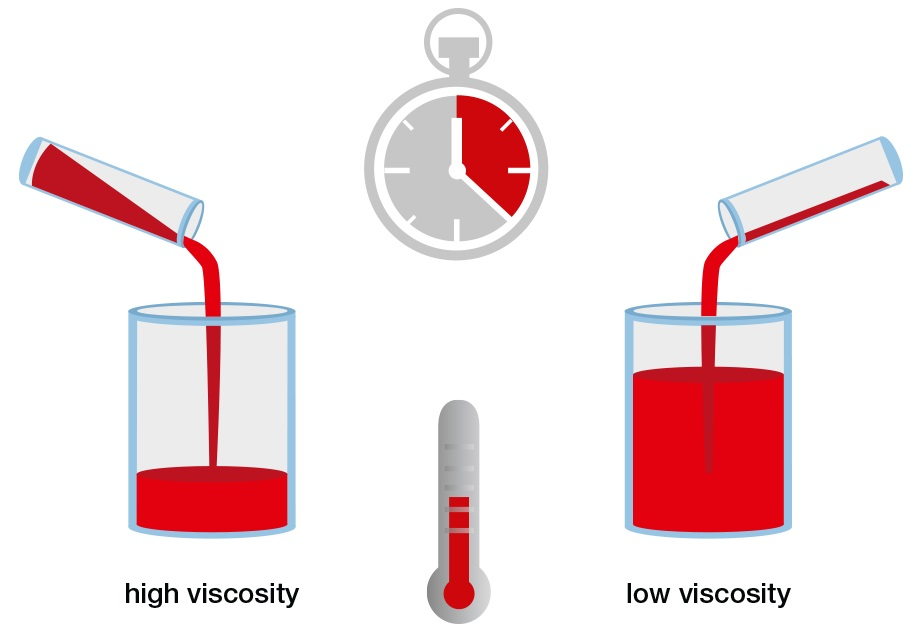Figure 1: Comparing high-viscosity fluid to low-viscosity fluid. At the same temperature, the former flows slower than the latter.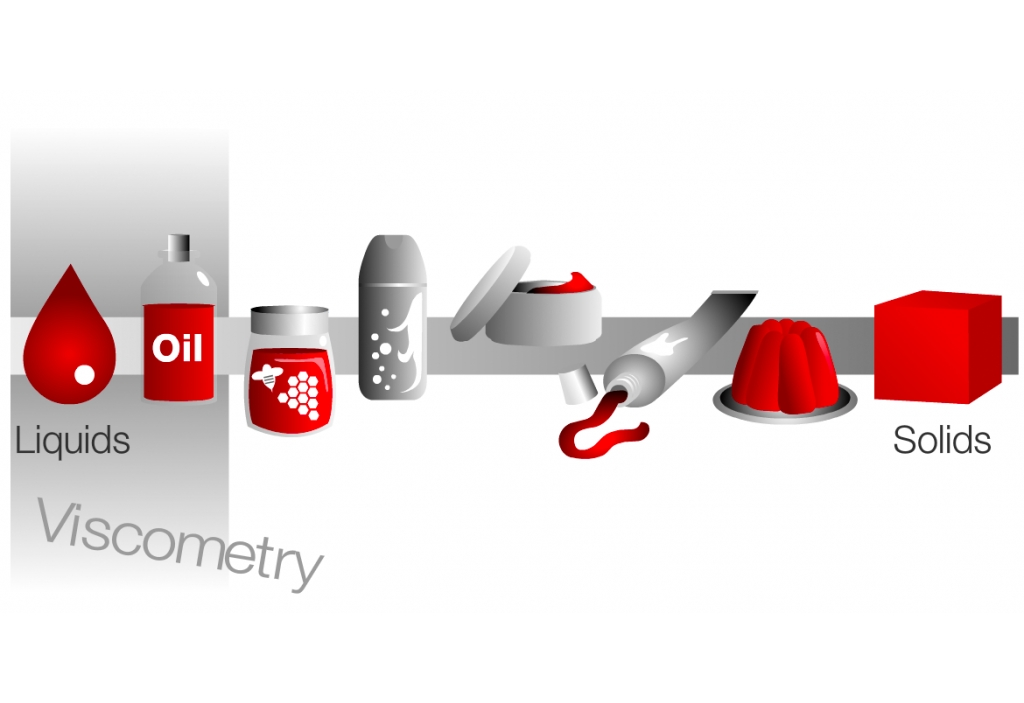Figure 2: From ideally viscous liquids to elastic solids. Viscoelastic materials in everyday life.

### The two plates model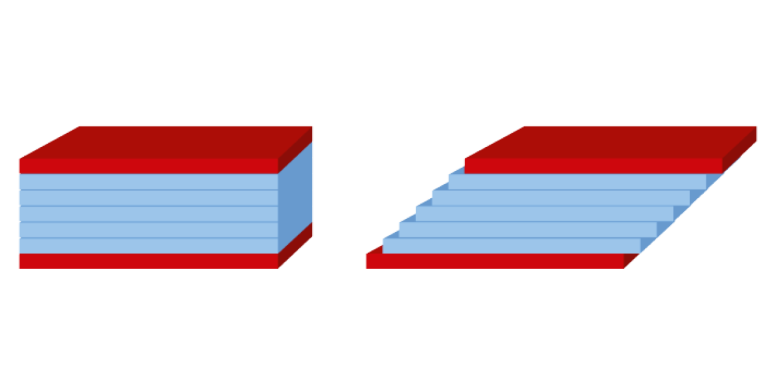Figure 3: The virtual viscous sandwich: the two-plates model. Laminar flow consisting of infinitesimally thin layers.

The two-plates model provides a mathematical description for viscosity. Think of a kind of sandwich: There are two plates with fluid placed in-between. The correct calculation of parameters related to viscosity depends on two criteria:

1. The fluid does not glide along the plates but is in good contact with them. Scientifically speaking, an adhesive force operates between fluid and plates.
2. The flow is laminar. It forms infinitesimally thin layers and no turbulence (i.e. vortices) occurs. You can picture laminar flow as a stack of paper sheets (or beer mats).

The lower plate does not move. The upper plate drifts aside very slowly and subjects the fluid to a stress, which is parallel to its surface: the shear stress (tau). The force applied to the upper plate (in newton) divided by this plate’s area (in square meters) defines the shear stress. Force/area results in the unit N/m², which is named pascal [Pa] after Blaise Pascal.

$$\tau = {F\over{A}} \left[N\over{m^2}\right]$$

Equation 1: Shear stress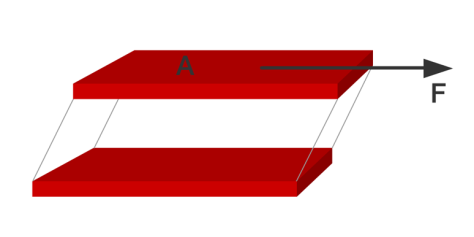Figure 4: Using the two-plates model to calculate the shear stress. Shear stress is the force moving the upper plate divided by the plate’s area.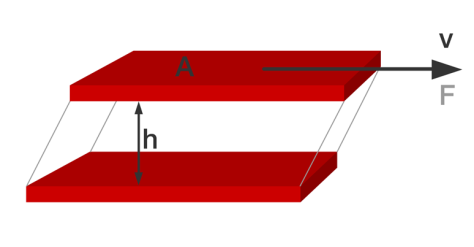Figure 5: Using the two-plates model to calculate the shear rate. Shear rate is the velocity of the moving plate divided by the distance between the plates.

The two-plates model allows for calculating another parameter: the shear rate (gamma-dot or also D).
The shear rate is the velocity of the upper plate (in meters per second) divided by the distance between the two plates (in meters). Its unit is [1/s] or reciprocal second [s-1].

$$\dot{\gamma}=(D=){v\over{h}} \left[{m\over{s \cdot m}}\right]\left[{1\over{s}}\right ]= [s^{-1}]$$

Equation 2: Shear rate

According to Newton’s Law, shear stress is viscosity times shear rate. Therefore, the viscosity (eta) is shear stress divided by shear rate. Only Newtonian liquids can be described by this simple relation.

$$\tau = {\eta}{\cdot} \dot{\gamma}\rightarrow {\eta} = {\tau \over \dot{\gamma}}[Pa \cdot {s}] = \left[{Pa \over {1 \over s}}\right]$$

Equation 3: Newton’s Law reformulated: Dynamic viscosity is shear stress divided by shear rate.

## What are Newtonian liquids?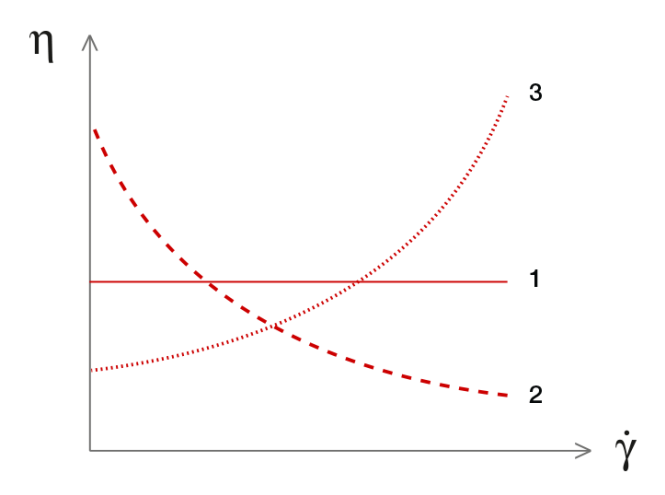Figure 6. Viscosity functions show dynamic viscosity vs. shear rate. Different types of flow behavior: Newtonian = ideally viscous (1) | Shear-thinning (2) | Shear-thickening (3)

If a fluid’s internal flow resistance is independent of the external force – i.e. the shear rate – acting upon the fluid, it is ideally viscous. Such fluids are named ‘Newtonian liquids’ after Sir Isaac Newton.

A viscosity function shows the viscosity over the shear rate. For a Newtonian liquid, this function is a straight line (curve 1); see figure 6.

Typical Newtonian liquids are water or salad oil.

If its viscosity changes with the shear rate, a liquid is non-Newtonian and – for exact definition – one has to specify the apparent viscosity.

Different shear-dependent fluids behave differently: For some, their viscosity decreases when the shear rate increases (curve 2 | e.g. yogurt), while for others the viscosity increases with increasing shear rate (curve 3 | e.g. starch solutions). The flow behavior of non-Newtonian liquids can be far more complex than these basic examples. To learn more consult “The Rheology Handbook.

Still, shear rate is not the only influencer. Three factors determine a substance’s flow behavior.

## What influences flow behavior?

1. The substance’s inner – molecular – structure. A highly viscous substance features tightly linked molecules. It resists deformation.
2. The shear rate or the shear stress, respectively, as external force. This includes all kinds of actions: wiping, or pushing, or pulling a substance, or – most commonly – gravity. The influence further depends on the strength and on the duration of the external force.
3. The ambient conditions: temperature and pressure. These parameters determine under which conditions a substance flows and which type of flow it develops. To measure viscosity, laminar flow is required.Figure 7. Three factors are responsible for a substance’s flow behavior: Inner molecular structure, outside forces acting on the material, and current ambient conditions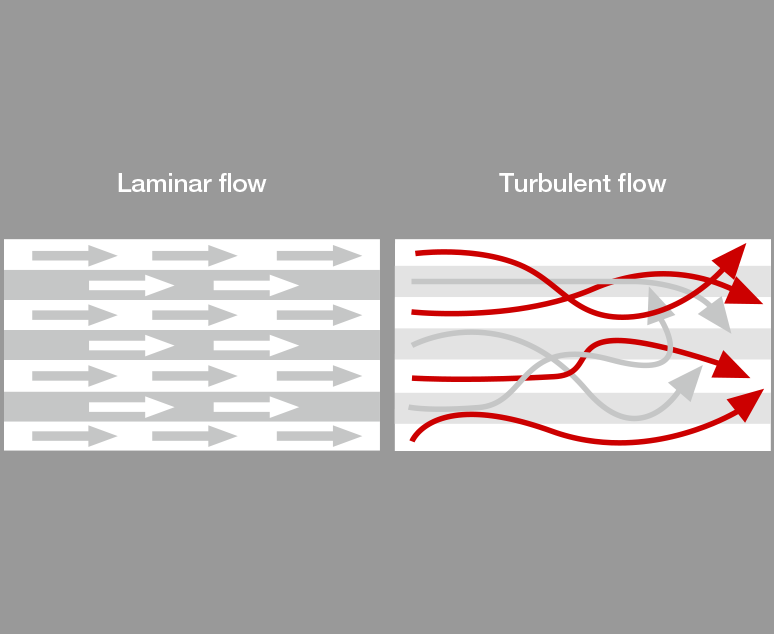Figure 8. In laminar flow, molecules move in orderly layers, while in turbulent flow they follow no pattern.

### Laminar or turbulent flow

Laminar flow means that the substance moves in imaginary thin layers. Molecules do not move from one layer to another, their movement forms a regular pattern.

Turbulent flow is not structured because molecules move at random. This leads to eddies and vortices and causes erroneous results during measurement. The viscosity appears higher than it is, because the test device falsely registers the molecules’ random behavior as higher flow resistance.

For example, submitting a fluid to a too high shear rate during the test can result in turbulent flow. That could happen if a glass capillary viscometer is too wide for the tested substance (i.e. the run times become too short) or if the spindle of a rotational viscometer turns too fast.

### Temperature

Apart from the shear rate, temperature strongly influences a fluid’s viscosity. A substance’s viscosity decreases with increasing temperature and vice versa. This inversely proportional relation applies to all substances.

Any change in temperature always influences viscosity, but for different fluids, the size of this influence varies. Certain fluids react with a 10 % increase in viscosity if temperature decreases by 1 °C.

A fluid’s viscosity strongly depends on its temperature. Even a 1 K (1 °C) temperature decrease can raise the viscosity by 10 % (Figure 10).

### Pressure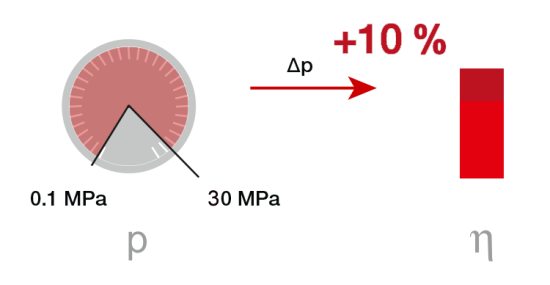Figure 11: Pressure has less influence on viscosity than temperature. Raising the pressure by a factor 300, results in a viscosity increase of approximately 10 %.

Normally, an increase in pressure causes a fluid’s viscosity to increase, too. However, fluids are not dramatically affected if the applied pressure is low or medium: liquids are almost non-compressible in this pressure range.

Most liquids react to a significantly altered pressure (from 0.1 MPa to 30 MPa) with a viscosity change of about 10 %. For comparison: This same change in viscosity is usually provoked by a minor temperature change of 1 K (1 °C).

In case the pressure goes up from 0.1 MPa to 200 MPa, the viscosity can rise to 3 to 7 times the original value. This applies to most low-molecular liquids. Highly viscous mineral oils react with a viscosity increase of times 20000 under identical circumstances.

For synthetic oil this pressure change can even result in a viscosity increase by a factor of up to 8 million. For example, lubricants in cogwheels or gears can be submitted to pressures of 1 GPa and higher.

1 bar = 0.1 MPa = 105 Pa = 105 N/m2
Equation 4. Conversion of pressure units.

In most liquids, pressure reduces the free volume in the internal structure, and thus limits the movability of molecules. Consequently, internal friction and viscosity increase.

#### How does water behave under pressure?

It is common knowledge that water has its maximum density at +4 °C. Another anomaly affects the flow behavior of water under pressure. For temperatures >+32 °C, the viscosity of water also increases with increasing pressure. If water is below +32 °C and pressure of up to 20 MPa is applied, the viscosity decreases while the pressure increases. The pressure destroys the structure of the three-dimensional network of hydrogen bridges.

## Types of viscosity

### Dynamic viscosity

The Greek symbol η (eta) represents the dynamic viscosity. In order to obtain dynamic viscosity (sometimes also known as shear viscosity), one has to reformulate Newton’s Law:

$$\tau = {\eta}{\cdot} \dot{\gamma}\rightarrow {\eta} = {\tau \over \dot{\gamma}}[Pa \cdot {s}] = \left[{Pa \over {1 \over s}}\right]$$

Equation 4: Newton's Law. How to derive dynamic viscosity.

SI (International System of Units) units:

• [Pa.s.] Pascal-second or [mPa.s] millipascal-second. 1 Pa.s = 1000 mPa.s

Other commonly used units:

• [P] Poise or [cP] centipoise (named after Jean Poiseuille): 1 P = 100 cP
• Relation between units: 1 cP = 1 mPa.s

Dynamic viscosity is preferably used in the fields of life science and research.

### Kinematic viscosity

The kinematic viscosity ν (ny) reflects the influence of the gravitational force on a substance’s flow. Dynamic viscosity divided by density ρ (rho), gives kinematic viscosity. As density is defined as mass per volume, gravity comes into the equation via the quantity mass.

$$\nu = {{\eta}\over \rho} \left[{m^{2}}\over s\right] \: \: \rho = {m \over V} \left[{kg}\over{m^{3}}\right]$$

Equation 5. Kinematic viscosity is dynamic viscosity divided by density. Gravitational influence is introduced through the mass, which is contained in density.

SI units:

• [ms2/s] square meters per second or [mm2/s] square millimeters per second
• 1 m2/s = 1 000 000 mm2/s

$$\left[{m^{2}}\over s\right] = \left[{Pa \cdot s} \over {kg \over {m^{3}}}\right] = \left[{N\over{m^{2}}\cdot s} \over {kg\over{m^{3}}}\right]$$

$$[N] = \left[{kg \cdot m} \over {s^{2}}\right]$$

$$\left[{m^{2}}\over {s}\right] = \left[{{kg \cdot m} \over {{s^{2}} \cdot {m^{2}}}} \cdot s \cdot {{m^{3}}\over kg}\right]$$

Equation 6. Deriving the SI unit of kinematic viscosity via the reformulated Newton’s Law.

Other commonly used units:

• [St] stokes or [cSt] centistokes (named after George G. Stokes).
• 1 St = 100 cSt
• 1 cSt = 1 mm2/s

Kinematic viscosity is widely used for all petrochemical fluids such as fuels or lube oils.

### Relative viscosity

Relative viscosity is a vital parameter when measuring dissolved polymers.

A polymer’s quality is strongly linked to its molar mass. The majority of polymers show a distinct relation between molar mass and viscosity. Therefore, in order to determine molar mass, one can measure viscosity instead. As a rule, the viscosity of polymer solutions increases with increasing molar mass. In fact, most polymer solutions are shear-dependent (i.e. non-Newtonian).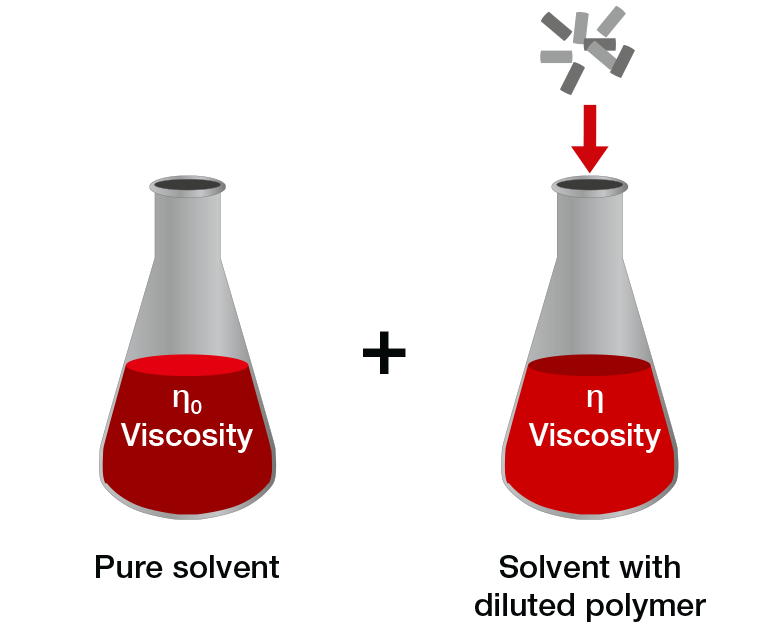Figure 11. Viscosity measurement of polymers in solution. First, measure the pure solvent and then a polymer solution of defined concentration.

However, in a range of sufficiently low shear rates, their behavior is Newtonian. Dividing the viscosity of the polymer solution (η) by the viscosity of the pure solvent (ηο) gives the relative viscosity with the dimensionless unit.

The relative viscosity ηr is the base for calculating other parameters, which are relevant in polymer quality control:

$${\eta _{r}}={{\eta}\over{\eta _{0}}}\left[1\right]$$

Equation 7: Relative viscosity is the viscosity of the polymer solution divided by the viscosity of pure solvent.

• Logarithmic viscosity number (also called inherent viscosity)
• Specific viscosity (also called relative viscosity increment)
• Reduced viscosity (also called viscosity number (VN) or Staudinger Function)
• Intrinsic viscosity (also called limiting viscosity number (LVN) or Staudinger Index)
• K-value
• Molar mass (molar mass is given as g/mol in most cases. It is defined as the mass of a certain substance divided by the amount of substance.)

### Apparent viscosity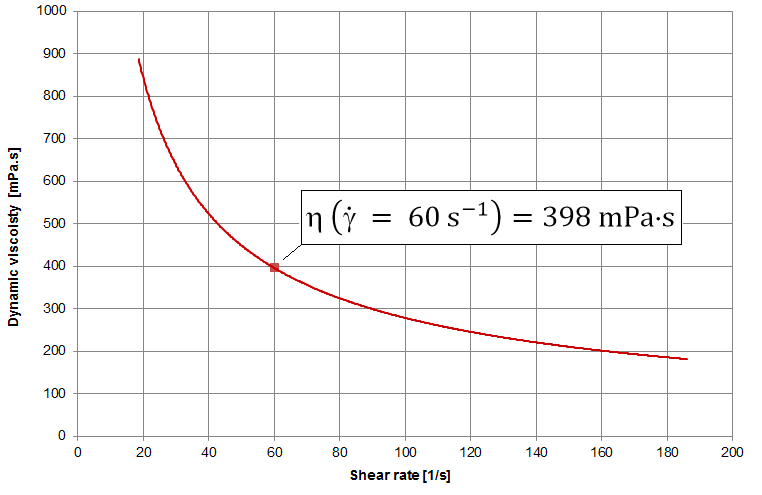Figure 12: Viscosity function of a shear-thinning fluid (e.g. shampoo). Apparent viscosity at a shear rate of 60⁻¹ shown as an example.

Ideally viscous or Newtonian fluids have constant viscosity for all shear rate values. For shear-dependent fluids, on the other hand, the viscosity varies. Therefore, you need to specify at which shear rate a viscosity value was determined. This is the “apparent viscosity” or “apparent shear viscosity”. Each apparent value is one point of the viscosity function (eta over shear rate).

Example:

$${\eta} (\dot{\gamma} = 60 {s^{-1}}) = 398 \: mPa \cdot s$$